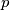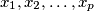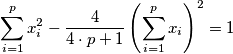### IMO Shortlist 1988 problem 22

Kvaliteta:
Avg: 0,0
Težina:
Avg: 0,0
Let$p$ be the product of two consecutive integers greater than 2. Show that there are no integers$x_1, x_2, \ldots, x_p$ satisfying the equationOR

Show that there are only two values of$p$ for which there are integers$x_1, x_2, \ldots, x_p$ satisfyingIzvor: Međunarodna matematička olimpijada, shortlist 1988